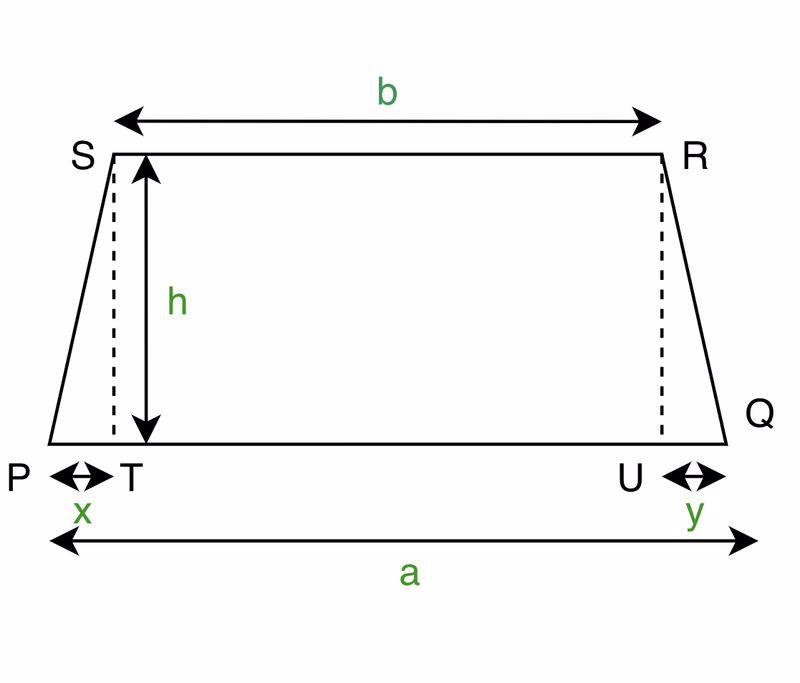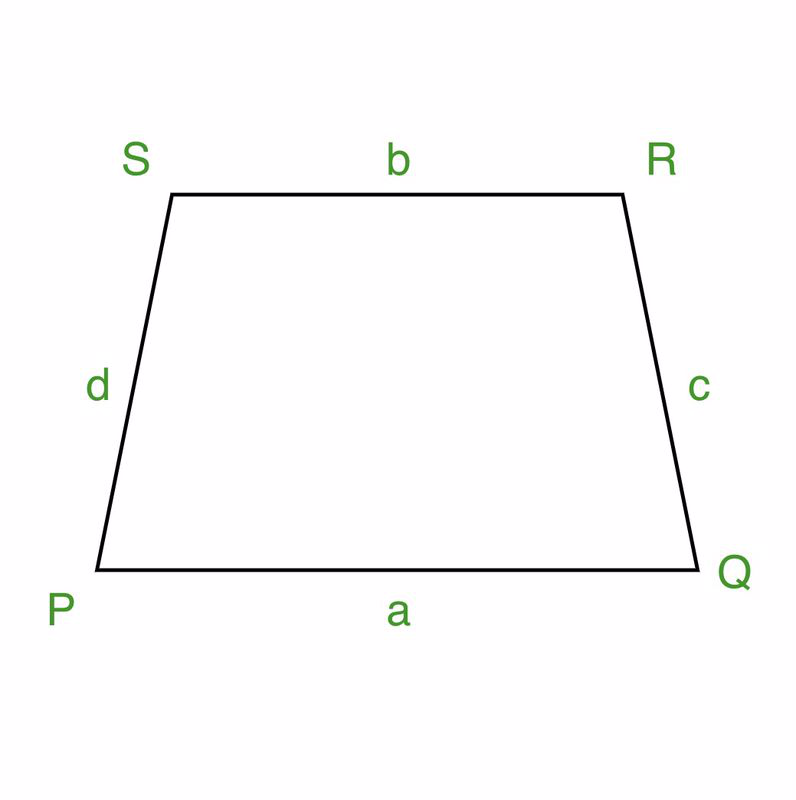Open in App
Not now

# Trapezoid Formula

• Last Updated : 30 May, 2022

Mensuration is a branch of geometrical mathematics which measures the geometrical quantities such as total surface area, lateral surface area, perimeter, length, breadth, and volume. 2D and 3D are the two different types of mensuration.

2D figures are formed by two or more straight lines or closed circular lines. These figures have no height or depth. Quantities such as area and perimeter can be determined for these figures. These are known as plane figures.

For Example circle, triangle, square, rectangle. 3D figures are formed by a number of surfaces or planes.  They have length, breadth, and height. These are solid figures. Total surface area, lateral surface area,, and volume can be determined for these figures. Example: cone, cylinder, cube.

### What is a Trapezoid?

It is a quadrilateral that has one pair of parallel sides and another set of non-parallel sides. The parallel sides are known as bases. The other two non-parallel sides are called lateral sides. Altitude or height is the shortest distance between the two parallel sides.

Types of Trapezoid

There are three types of trapezoids. They are

1. Isosceles Trapezoid: The lengths of non-parallel sides are equal.
2. Right-angled Trapezoid: It has a set of right angles.
3. Scalene Trapezoid: No sides are equal.

Properties of a Trapezoid

• A Trapezoid has only one pair of parallel sides.
• The Sum of all angles of a Trapezoid is 360o.
• For a Isosceles Trapezoid, both base angles are congruent , non parallel sides are congruent and diagonals are congruent.
• The median is parallel to both the bases and is the average length of the bases.
• The adjacent angles sum to 180o.

### Trapezoid Formulas

The formula of Trapezoid consists of the area and perimeter. Now let’s look at how the formulas are derived.

Area of TrapezoidLet us consider a trapezoid  PQRS, SR and PQ are parallel sides and ST is the shortest distance between them which is perpendicular and is denoted with h. Let‘s divide the trapezoid into segments. That gives us two triangles PTS and UQR and a rectangle TURS. Now if we find the areas of all three and sum them, we get the area of the Trapezoid.

In writing the area of triangle, PTS = 1/2 (base × height)

Area of triangle, PTS = 1/2 (PT × ST)

= 1/2 (xh)                     …..(1)

On writing the area of rectangle TURS = length × breadth

Area of rectangle TURS = bh                     …..(2)

In writing the area of triangle UQR = 1/2 (base × height)

Area of triangle UQR = 1/2 ( yh)                     …..(3)

In summing up the three areas i.e, equations (1), (2), and (3), we get the area of the trapezium.

On adding all the three equations,

Area of trapezium = 1/2 (xh) + bh + 1/2 ( yh)

on taking 1/2 (h) common, we can write the equation as

Area of trapezium = (1/2) h (x + 2b + y)

Area of trapezium = (1/2) h ( x + b + b + y)

From the figure, we can observe that summing x, b, and y gives a. So,

Area of trapezium = (1/2) × h × (a+b)

or

Area of trapezium = (1/2) × (height) × (sum of the parallel sides)

Hence, the area of the trapezium is half the product of the height and the sum of parallel sides.

Perimeter of TrapezoidFor finding the perimeter, the lengths of all the sides are to be added.

Perimeter of the trapezoid = Sum of lengths of parallel and non-parallel sides

or

Perimeter of trapezoid = PQ + RS + QR + PS

or

Perimeter of trapezoid = a + b + c + d

Hence, the Perimeter of a trapezoid is the sum of lengths of parallel sides and the sum of lengths of non-parallel sides.

### Sample Questions

Question 1: Find the area of trapezoid if the bases are 10 cm and 16 cm. The shortest distance between the parallel sides is 8 cm.

Given lengths of bases, a= 10 cm and  b= 16cm, height h = 8cm.

Area of trapezium = (1/2) h (a+b)

= (1/2) 8 (10+16)

= 140 cm2

Question 2: If the area of a trapezoid is given as 240 cm2 and the sum of lengths of parallel sides is given as 30 cm, find the height of the trapezoid.

Given, Area = 240cm2 ,(a+b) = 30cm

Area of trapezium = (1/2) h (a+b)

240  = (1/2) h (30)

h = (240 × 2) / 30

= 16 cm

Hence, the height of trapezoid is 16 cm.

Question 3: Find the perimeter of the trapezoid if the lengths of the sides are 15cm, 6cm, 12 cm, and 8 cm respectively.

Given, a= 15 cm, b= 6 cm, c= 12 cm, and d= 8 cm.

Perimeter of trapezoid = sum of lengths of parallel sides and sum of lengths of non parallel sides.

= a + b + c + d

= 15+6+12+8

= 41 cm

Question 4: If the perimeter of a trapezium is 48 cm and the sum of parallel sides is 26 cm and the length of the Third side is 10 cm. Find the length of the fourth side.

Given, a+b = 26 cm , c =10 cm and Perimeter = 48 cm

The perimeter of the trapezoid = the sum of lengths of parallel sides and the sum of lengths of nonparallel sides.

Perimeter of trapezoid = a + b + c + d

48 = 26 + 10 + d

d = 48- 26 – 10

= 12 cm

Hence the length of the fourth side is 12 cm.

Question 5: If the length of a parallel side is greater than the other by 6 cm and the area of a trapezoid is 240 cm2. Find the lengths of the parallel sides If the shortest distance between the parallel sides is 14 cm.

Given, Area = 240 cm2, and Height = 14 cm.

Let a parallel side a = x ,

According to the given condition,

b = x + 6

Area of trapezium = (1/2) h (a+b)

240 = (1/2) (14) (x + x + 6)

(240×2) / 14 = 2x + 6

2x = 34.28 – 6

2x = 28.28

x = 14.14

Hence a = 14.14 cm

b = x + 6

b = 14.14 + 6

b = 20.14 cm

Hence 14.14 cm and 20.14 cm are the lengths of the parallel sides.

My Personal Notes arrow_drop_up
Related Articles